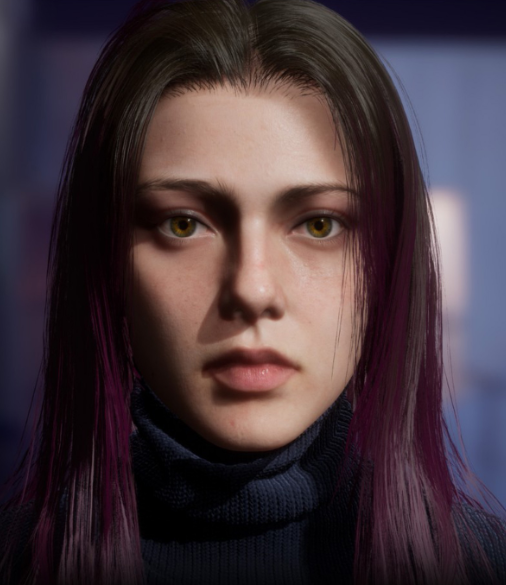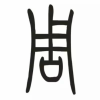# MarvelousDesigner全方位布料造型教程

#### ￥300.00 / ￥599.00 / VIP ￥280.00•     MD基础界面
•     基础版型
•     布料属性讲解
•     窗帘
•     床上用品-A
•     床上用品-B
•     床上用品-C
•     床上用品-D
•     小花枕头
•     宽松牛仔裤-A
•     宽松牛仔裤-B
•     宽松牛仔裤-C
•     皮裤-A
•     皮裤-B
•     松紧短裤制作
•     小腿牛仔裤-A
•     小腿牛仔裤-B
•     垃圾袋
•     阿拉伯头巾
•     阿拉伯围巾
•     阿拉伯围巾-A
•     面巾
•     巫师帽
•     紧身衣-A
•     紧身衣-B
•     紧身衣-C
•     运动裤
•     运动卫衣
•     百褶裙-A
•     百褶裙-B
•     花瓣裙
•     打结衣服-A
•     打结衣服-B
•     打结衣服-C
•     绑带衣服
•     西服-A
•     西服-B
•     西服-C
•     西裤
•     衬衣
•     领带
•     罗马服饰-女-A
•     罗马服饰-女-B
•     罗马服饰-女-C
•     罗马服饰-女-D
•     罗马服饰-女-E
•     皮大衣-A
•     皮大衣-B
•     青年皮大衣-A
•     青年皮大衣-B
•     大衣-A
•     大衣-B
•     羽绒服-A
•     羽绒服-B
•     羽绒服-C
•     古装-男-A
•     古装-男-B
•     古装-男-C
•     古装-男-D
•     古装-男-E
•     古装-男-F
•     古装-男-G
•     古装-男-H
•     古装-男-束腰
•     汉服-女-A
•     汉服-女-B
•     汉服-女-C
•     汉服-女-D
•     汉服-女-E
•     洛可可-女-A
•     洛可可-女-B
•     洛可可-女-C
•     洛可可-女-D
•     洛丽塔-A
•     洛丽塔-B
•     洛丽塔-C
•     洛丽塔-D
•     洛丽塔-E
•     洛丽塔-F
•     洛丽塔-G
•     洛丽塔-H
•     洛丽塔-I
•     洛丽塔-J
•     洛丽塔-K
•     洛丽塔-L
•     木乃伊-A
•     木乃伊-B
•     巫师袍子-A
•     巫师袍子-B
•     巫师袍子-C
•     巫师袍子-D
•     巫师袍子-E
•     巫师袍子-F
•     巫师袍子-G
•     巫师袍子-H
•     巫师袍子-I
•     POSE-女汉服-A
•     POSE-女汉服-B
•     POSE-女汉服-C
•     POSE-女汉服-D
•     POSE-女汉服-E
•     POSE-女汉服-F
•     POSE-女汉服-G
•     背包-A
•     背包-B
•     背包-C
•     背包-D
•     背包-E
•     背包-F
•     背包-G
•     背包-H
•     工装-A
•     工装-B
•     工装-C
•     工装-D
•     工装-E
•     工装-F
•     工装-G
•     工装-H
•     max-布线
•     maya-UV-布线-A
•     maya-UV-布线-B
• ### 5条评价提交评价

1.娱乐至死 好评

老师是不是少了一节课啊 罗马服饰那里

•课程已遗失，如有问题请在售后群联系讲师

2.血树残株 好评

3.做个安静的艺术人 好评

怎么看了啊，

•提示什么

4.世间哪有奇遇？ 好评

实战性很强的一套课程，讲师后面还会女装出镜，冲了。

•感谢你的支持和认可

5.山不周 好评

实战性很强的一套课程，不错。

•厉害了

### 我的提问提交问答

下载地址

请购买后查看

售后QQ群

请购买后查看

•#### 在线客服

##### 在线咨询
•#### 联系电话

##### 联系电话

023-63249883

•#### 公众号

##### 微信关注•#### 发送邮件

•